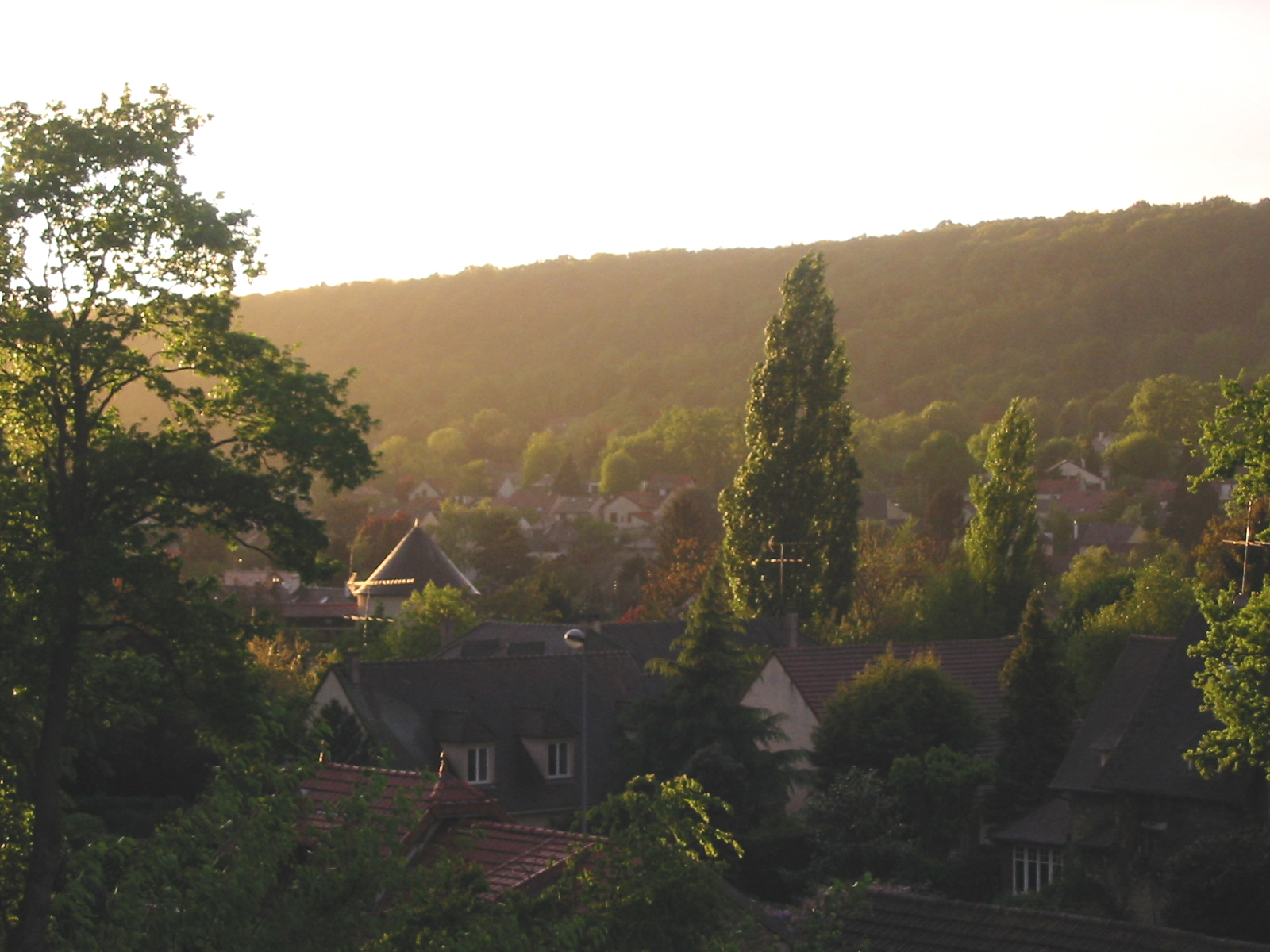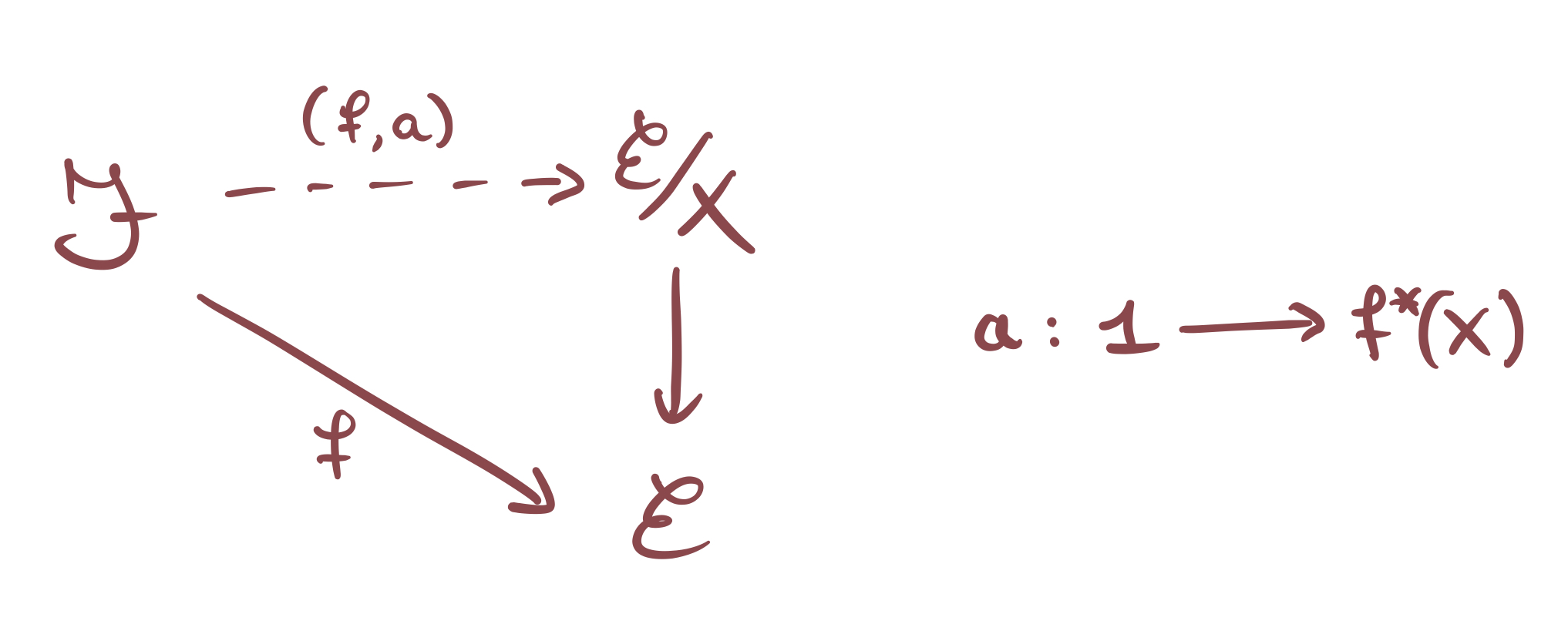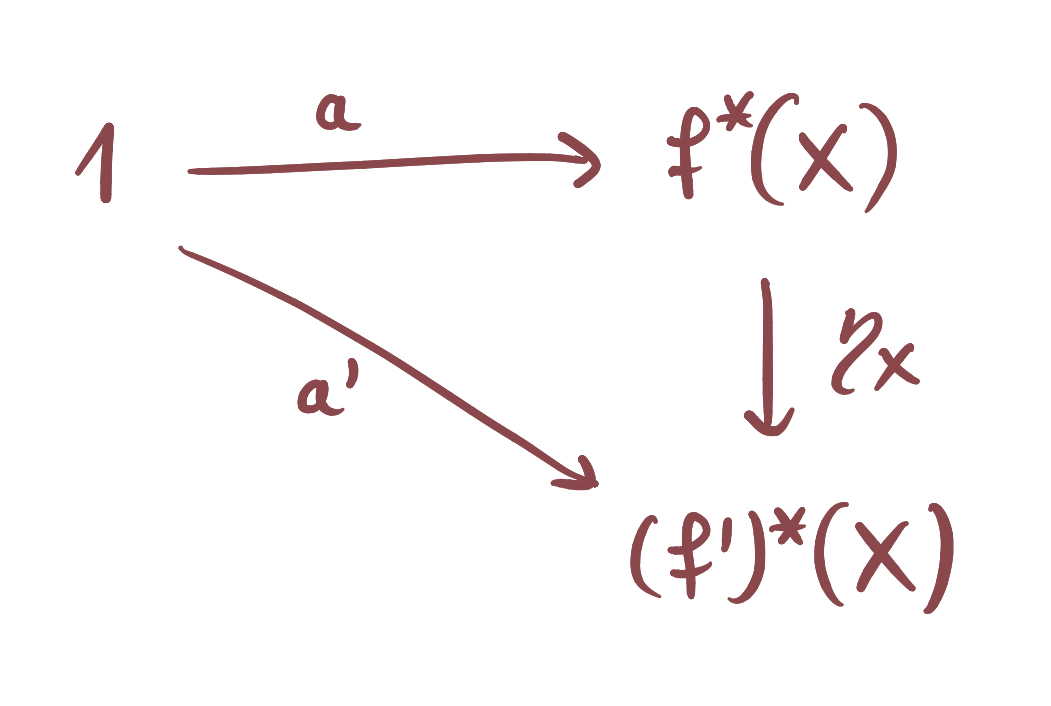by Jens Hemelaer

One of the main results about sheaves on a topological space $$X$$​​ is that they correspond to étale spaces over $$X$$​​, so to local homeomorphisms $$Y \to X$$​​. This can be turned into an equivalence of categories between the category of sheaves on $$X$$​​ and the category of local homeomorphisms $$Y \to X.$$​​ The generalization of this statement to toposes (rather than topological spaces) is well-known among topos theorists, but it took me a really long time to find this statement in the literature. It turns out the result was in SGA4… I could have seen this coming.The result from SGA4 is much more general (no surprises here) and gives a method of determining the geometric morphisms $$\mathcal{F} \to \mathcal{E}/X$$ in terms of geometric morphisms $$\mathcal{F} \to \mathcal{E}$$. Here $$\mathcal{E}/X$$ denotes the slice topos over the object $$X$$ in $$\mathcal{E}$$. It’s a really interesting result, and with this blogpost, I will try to make it a bit more well-known.

After stating the result in full detail, we will see how to make sense of the result using Yoneda’s notation for discrete opfibrations. Then we derive from the SGA4 result the correspondence between sheaves and local homeomorphisms, for arbitrary toposes (this is left as an exercise in SGA4, because all the hard work is in the more general result).

A detailed proof of the SGA4 result is given in the accompanying pdf here. The method is the same as in SGA4.

While writing this blog post, I was influenced and encouraged by interesting discussions about this with Morgan Rogers and Steve Vickers. I also would like to mention the recent paper by Caramello and Zanfa, which features a short proof of the correspondence between sheaves and local homeomorphisms over a Grothendieck topos (see Lemma 6.1.2), and puts it in a much wider context.

EDIT (November 2021): The result from SGA4 that I discuss here also appears as Lemma 4.59 in the paper “Denseness conditions, morphisms and equivalences of toposes” (2020) by Olivia Caramello. So the result from SGA4 has some applications in recent topos theory literature. It is very likely that I first read the result in Caramello’s paper, but then forgot about it until I found it in SGA4.

## Statement in SGA4

The statement in SGA4 says that the geometric morphisms $$g : \mathcal{F} \to \mathcal{E}/X$$​​ correspond to the pairs $$(f,a)$$​​ where $$f : \mathcal{F} \to \mathcal{E}$$​​ is a geometric morphism and $$a : 1 \to f^*(X)$$​​ is a global element of $$f^*(X)$$​​​.Further, suppose that $$g,g' : \mathcal{F} \to \mathcal{E}/X$$​​ are two geometric morphisms corresponding to the pairs $$(f,a)$$​​ respectively $$(f',a')$$​​. Then the geometric transformations $$g \to g'$$​​ correspond to the natural transformations $$\eta : f^* \to (f')^*$$​​ such that $$\eta_X \circ a = a'$$​​​.Here we use the convention that a geometric morphism $$g \to g'$$ is a natural transformation $$g^* \to (g')^*$$. Nowadays, this is the standard convention, but unfortunately SGA4 uses the opposite convention that a morphism $$g \to g'$$ is a natural transformation $$g_* \to (g')_*$$ or equivalently a natural transformation $$(g')^* \to g^*$$.

Before I forget, I should give the full reference: the general result I am referring to is SGA4, Tome 1, Exposé IV, Proposition 5.12. The description of geometric morphisms $$\mathcal{E}/Y \to \mathcal{E}/X$$​ over $$\mathcal{E}$$​ is a special case; so it is left as Exercise 5.14 in the same section. SGA4 has multiple authors; this particular Exposé is attributed to Grothendieck and Verdier. The relevant part of SGA4 was pointed out to me by the reference section of the nLab page on slice toposes.

I think one of the reasons why I didn’t find the relevant section in SGA4 earlier, is that I typically used the non-searchable scans. From now on, I want to use the searchable pdf versions, put together by Laszlo (Tome 1, Tome 2, Tome 3). An overview of Grothendieck’s writings is made on Carmona’s website Thèmes pour une Harmonie.

## Yoneda’s notation and discrete opfibrations

I spelled out the result in detail above, but in order to make it easy to remember we have to “condense” the result in simpler but more abstract terms. Then the result is this:

$$\mathbf{Geom}(\mathcal{F},\mathcal{E}/X) ~\simeq~ \int^{f \in \mathbf{Geom}(\mathcal{F},\mathcal{E})} \mathrm{Hom}_\mathcal{F}(1,f^*(X)).$$​​

Here $$\mathbf{Geom}(\mathcal{T},\mathcal{T}')$$​​ denotes the category of geometric morphisms from $$\mathcal{T}$$​​ to $$\mathcal{T'}$$​​ (and geometric transformations between them), for any two toposes $$\mathcal{T}$$​​ and $$\mathcal{T}'$$​​​.

The idea behind the notation with integral sign is to look at the projection functor $$\xi : \mathbf{Geom}(\mathcal{F},\mathcal{E}/X) \to \mathbf{Geom}(\mathcal{F},\mathcal{E})$$​​​ given by composition with the projection $$\mathcal{E}/X \to \mathcal{E}$$​​​. Then the SGA4 result mentioned above says that $$\xi$$​​​ is a discrete opfibration on $$\mathbf{Geom}(\mathcal{F},\mathcal{E})$$​​​; more precisely, it is the discrete opfibration corresponding to the functor $$\mathbf{Geom}(\mathcal{F},\mathcal{E}) \to \mathbf{Sets}$$​​​ that sends $$f$$​​​ to $$\mathrm{Hom}_{\mathcal{F}}(1,f^*(X))$$​​​.

The notation with integral signs for discrete (op)fibrations is due to Yoneda. For a functor $$F : \mathcal{C} \to \mathbf{Sets}$$​, there are two possible notations for the discrete opfibration associated to $$F$$​, namely $$\int^{c \in \mathcal{C}} F(c)$$​ or the shorter $$\int^\mathcal{C} F$$​​. The result above is a good example of a situation where the longer notation (with a “variable”) makes things clearer.Above: Gare du Nord in the fifties, where the Yoneda Lemma was born.

Spelled out, $$\int^{c \in \mathcal{C}} F(c)$$ is the category with

• as objects the pairs $$(c,a)$$ with $$c$$ an object in $$\mathcal{C}$$ and $$a \in F(c)$$ an element;
• as morphisms $$(c,a) \to (c',a')$$ the morphisms $$h : c \to c'$$ in $$\mathcal{C}$$ such that $$F(h)(a) = a'$$.

Sometimes, the notation $$\int F$$ is used, but this is ambiguous. There is a dual notation $$\int_\mathcal{D} G$$ to denote the discrete fibration associated to a contravariant functor $$G : \mathcal{D}^\mathrm{op} \to \mathbf{Sets}$$. So when you see $$\int F$$ it can mean both $$\int^\mathcal{C} F$$ or $$\int_{\mathcal{C}^\mathrm{op}} F$$, and these are different (the morphisms go in opposite directions).

## Morphisms between slice toposes

As a special case of the result above, we can look at geometric morphisms between two slice toposes (which is the result I was originally interested in).

Let $$\mathcal{E}$$ be a (Grothendieck) topos and let $$X$$ and $$Y$$ be objects in $$\mathcal{E}$$. We consider the slice toposes $$\mathcal{E}/X$$ and $$\mathcal{E}/Y$$, and we write $$x : \mathcal{E}/X \to \mathcal{E}$$ respectively $$y : \mathcal{E}/Y \to \mathcal{E}$$ for the projection geometric morphisms.For every $$\phi : X \to Y$$ in $$\mathcal{E}$$ there is a corresponding induced geometric morphism $$f: \mathcal{E}/X \to \mathcal{E}/Y$$ such that $$y \circ f \simeq x$$. This is the geometric morphism with inverse image functor $$f^*$$ given by pullback along $$\phi : X \to Y$$.

The question is now: if $$f : \mathcal{E}/X \to \mathcal{E}/Y$$ is a geometric morphism with $$y \circ f \simeq x$$, then is it always induced by some map $$\phi : X \to Y$$ in $$\mathcal{E}$$ ? The answer turns out to be yes!

Why is this important? The reason is that, by definition, local homeomorphisms with codomain $$\mathcal{E}$$ are precisely the geometric morphisms of the form $$\mathcal{E}/X \to \mathcal{E}$$ for some object $$X$$ in $$\mathcal{E}$$. So by definition, “sheaves” (i.e. objects of $$\mathcal{E}$$) correspond to local homeomorphisms to $$\mathcal{E}$$. But if we want to turn this into an equivalence of categories, we need that morphisms $$\phi : X \to Y$$ in $$\mathcal{E}$$ correspond precisely to the geometric morphisms $$\mathcal{E}/X \to \mathcal{E}/Y$$ over $$\mathcal{E}$$​.

In the special case where $$\mathcal{E} = \mathbf{Sh}(L)$$​​​​​​​ for some topological space or locale $$L$$​​​​​, we know that the local homeomorphisms with codomain $$\mathbf{Sh}(L)$$​​​​​ are precisely the geometric morphisms $$\mathbf{Sh}(L') \to \mathbf{Sh}(L)$$​​​​​ induced by local homeomorphisms $$L' \to L.$$​​​​​ Starting from this, the equivalence of categories between sheaves on $$\mathbf{Sh}(L)$$​​​​​ and local homeomorphisms to $$L$$​​​​​ then follows from the general correspondence between geometric morphisms $$\mathcal{E}/X \to \mathcal{E}/Y$$​​​​​ over $$\mathcal{E}$$​​​​​ and maps $$X \to Y$$​​​​​ in $$\mathcal{E}.$$​​​​​​​​​

## Morphisms between slice toposes: proof

To prove the correspondence, we use the result from SGA4 and some general results about discrete opfibrations.

We saw that $$\mathbf{Geom}(\mathcal{E}/X, \mathcal{E}/Y) ~\simeq~ \int^{h \in \mathbf{Geom}(\mathcal{E}/X,\mathcal{E})} \mathrm{Hom}_{\mathcal{E}/X}(1,h^*(X))$$. I changed the “variable” to $$h$$ rather than $$f$$ because we are already using $$f$$ to denote a map $$\mathcal{E}/X \to \mathcal{E}/Y$$.

We are interested in the category $$\mathbf{Geom}_\mathcal{E}(\mathcal{E}/X,\mathcal{E}/Y)$$ consisting of the geometric morphisms $$f : \mathcal{E}/X \to \mathcal{E}/Y$$ such that $$y \circ f \simeq x$$, and as morphisms the geometric transformations $$\eta : f \to f'$$ such that $$y \circ \eta$$ is the identity geometric transformation from $$x$$ to itself.

In the terminology from discrete opfibrations, $$\mathbf{Geom}_\mathcal{E}(\mathcal{E}/X,\mathcal{E}/Y)$$ is precisely the fiber of the discrete opfibration

$\mathbf{Geom}(\mathcal{E}/X, \mathcal{E}/Y) \longrightarrow \mathbf{Geom}(\mathcal{E}/X, \mathcal{E})$

above the geometric morphism $$x : \mathcal{E}/X \to \mathcal{E}$$​. Now we can use a general procedure. For a discrete opfibration $$\int^{c \in \mathcal{C}} F(c)$$​, the fiber above an object $$c_0$$​ of $$\mathcal{C}$$​ is precisely given by $$F(c_0)$$​. More precisely, the objects of the fiber are the elements of $$F(c_0)$$​, and there are no morphisms (other than the identity morphisms). This means that the fibers are all discrete categories, as the name ‘discrete opfibration’ suggests.

If we apply this to our case, we see that the fiber $$\mathbf{Geom}_\mathcal{E}(\mathcal{E}/X,\mathcal{E}/Y)$$ is the discrete category corresponding to the set $$\mathrm{Hom}_{\mathcal{E}/X}(1,x^*(Y))$$. The functor $$x^*$$ has a left adjoint $$x_!$$, so we compute

$\mathbf{Geom}_\mathcal{E}(\mathcal{E}/X,\mathcal{E}/Y) ~\simeq~ \mathrm{Hom}_{\mathcal{E}/X}(1,x^*(Y)) ~\simeq~ \mathrm{Hom}_\mathcal{E}(x_!(1),Y).$

The functor $$x_!$$ sends an object $$\alpha : A \to X$$ of $$\mathcal{E}/X$$ to the object $$A$$ of $$\mathcal{E}$$. The terminal object $$1$$ in $$\mathcal{E}/X$$ is given by the identity $$X \to X$$, so we see that $$x_!(1) \simeq X$$. In this way, we find:

$\mathrm{Hom}_\mathcal{E}(x_!(1),Y) ~\simeq~ \mathrm{Hom}_\mathcal{E}(X,Y).$

We conclude that:

$\mathbf{Geom}_\mathcal{E}(\mathcal{E}/X,\mathcal{E}/Y) ~\simeq~ \mathrm{Hom}_\mathcal{E}(X,Y).$So geometric morphisms $$f : \mathcal{E}/X \to \mathcal{E}/Y$$ over $$\mathcal{E}$$ correspond precisely to the maps $$X \to Y$$ in $$\mathcal{E}$$. Further, we also get a lot of information about the geometric transformations. Suppose that $$f, f' : \mathcal{E}/X \to \mathcal{E}/Y$$ are two geometric morphisms over $$\mathcal{E}$$, corresponding to the maps $$\phi, \phi' : X \to Y$$ in $$\mathcal{E}$$. Then there is at most one geometric transformation $$\eta : f \to f'$$ such that $$y \circ f$$ is the identity geometric transformation on $$x$$. This geometric transformation is always an isomorphism, and it exists if and only if $$\phi = \phi'$$​.

A direct proof is also possible, see e.g. the recent paper here by Caramello and Zanfa. There the equivalence $$\mathbf{Geom}_\mathcal{E}(\mathcal{E}/X,\mathcal{E}/Y) \simeq \mathrm{Hom}_\mathcal{E}(X,Y)$$​ appears as Lemma 6.1.2. The underlying idea of the proof is similar to the proof of the more general statement in SGA4.

## The situation for $$(\infty,1)$$​-toposes

With $$\mathbf{Topos}_\mathcal{E}^\text{ét}$$​​​ we denote the 2-category with as objects the toposes of the form $$\mathcal{E}/X$$​​​ (equipped with their projection geometric morphism to $$\mathcal{E}$$​​​), as morphisms the geometric morphisms over $$\mathcal{E}$$​​​, and as 2-morphisms the geometric transformations $$f \to f'$$​​​ relative over $$\mathcal{E}$$​​​, i.e. geometric transformations such that after postcomposing with the projection you get the identity geometric transformation.

Then we can summarize the result proved in the previous section as $$\mathbf{Topos}_\mathcal{E}^\text{ét} \simeq \mathcal{E}$$​​. In particular, $$\mathbf{Topos}_\mathcal{E}^\text{ét}$$​​ is equivalent to a 1-category, so there are essentially no nontrivial 2-morphisms.

A similar equivalence $$\mathbf{Topos}^\text{ét}_\mathcal{E} \simeq \mathcal{E}$$​​ appears for $$(\infty,1)$$​​-toposes in Lurie’s “Higher Topos Theory” (Remark 6.3.5.10). In “Higher Orbifolds and Deligne-Mumford Stacks as Structured Infinity-Topoi” (Corollary 3.2.1) Lurie’s result is used by Carchedi to show that the result holds for $$n$$​​-toposes for arbitrary $$n$$​​, in particular for (1-)toposes.

I thought for some time that this proof via higher toposes was the only one that appears in detail in the literature, and I mentioned this during my talk at Toposes Online. This is before I noticed the proof in SGA4, and before Caramello and Zanfa put their manuscript on the arXiv.